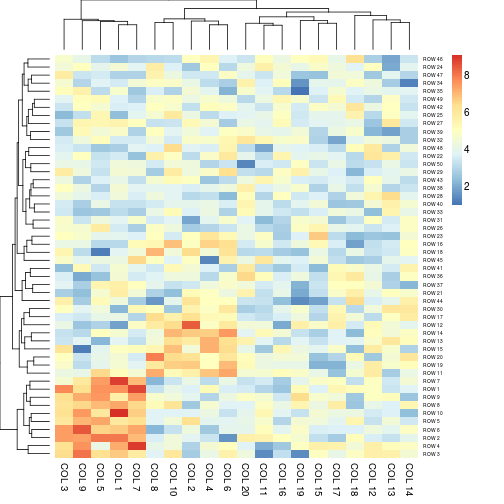# Annotated heatmaps

```  # Generate random datan <- 50; p <- 20
x <- abs(rmatrix(n, p, rnorm, mean=4, sd=1))
x[1:10, seq(1, 10, 2)] <- x[1:10, seq(1, 10, 2)] + 3
x[11:20, seq(2, 10, 2)] <- x[11:20, seq(2, 10, 2)] + 2
rownames(x) <- paste("ROW", 1:n)
colnames(x) <- paste("COL", 1:p)
## Scalingaheatmap(x, scale = "row")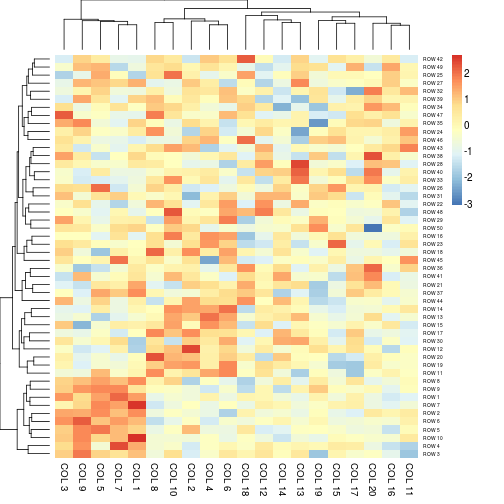aheatmap(x, scale = "col") # partially matched to 'column'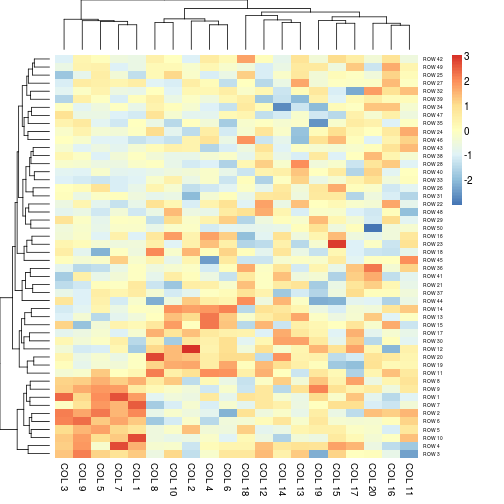aheatmap(x, scale = "r1") # each row sum up to 1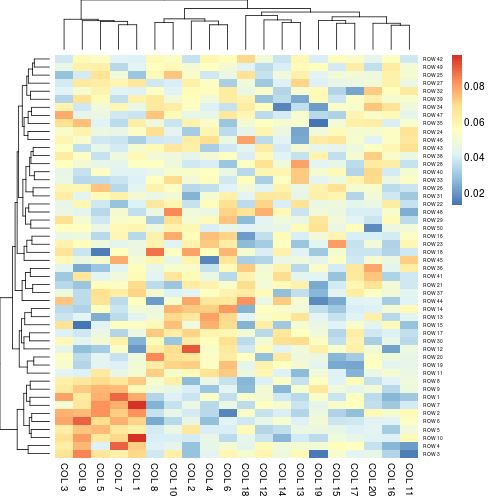aheatmap(x, scale = "c1") # each colum sum up to 1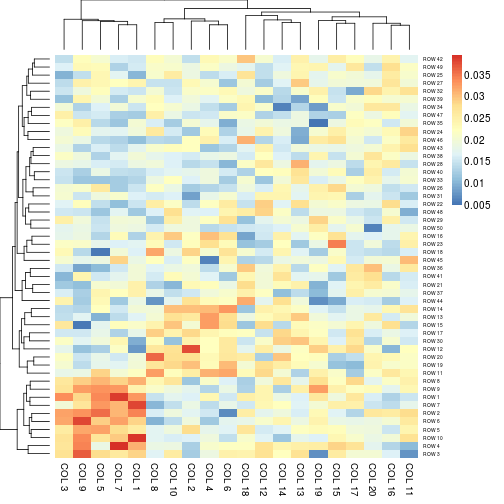## Heatmap colorsaheatmap(x, color = colorRampPalette(c("navy", "white", "firebrick3"))(50))# color specification as an integer: use R basic colorsaheatmap(x, color = 1L)# color specification as a negative integer: use reverse basic paletteaheatmap(x, color = -1L)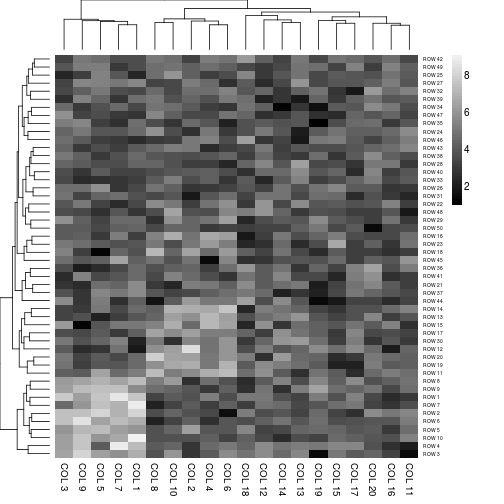# color specification as a numeric: use HCL coloraheatmap(x, color = 1)# do not cluster the rowsaheatmap(x, Rowv = NA)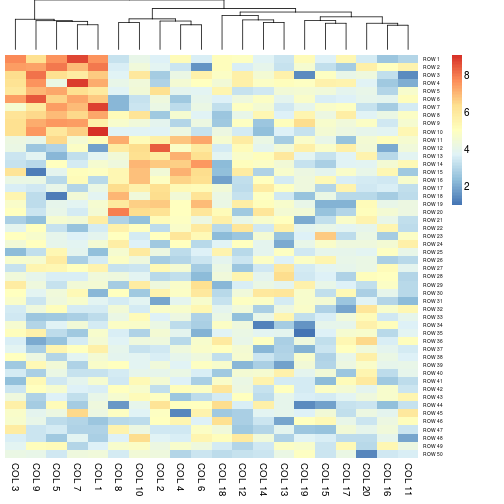# no heatmap legendaheatmap(x, legend = FALSE)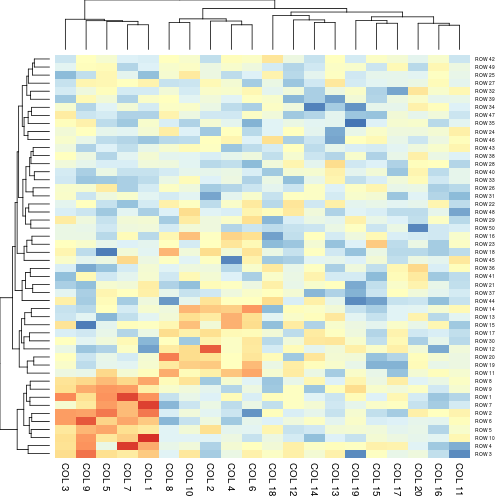# cell and font sizeaheatmap(x, cellwidth = 10, cellheight = 5)# directly write into a fileaheatmap(x, cellwidth = 15, cellheight = 12, fontsize = 8, filename = "aheatmap.pdf")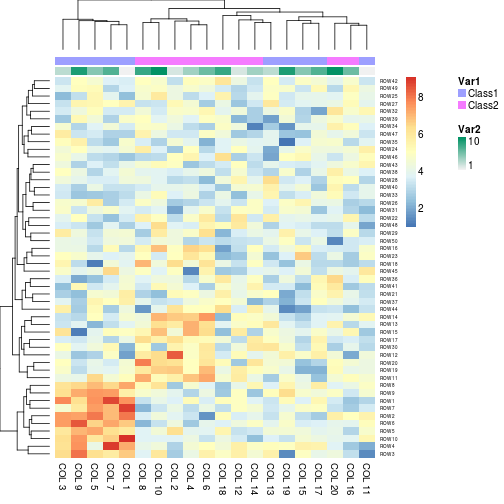aheatmap(x, annCol = annotation, annLegend = FALSE)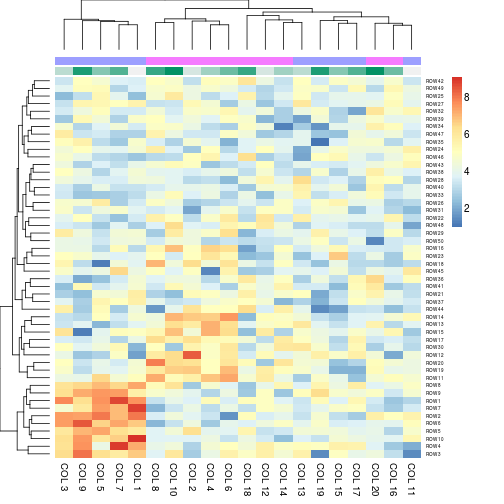# Specify colorsVar1 = c("navy", "darkgreen")# Specifying clustering from distance matrixdrows = dist(x, method = "minkowski")
aheatmap(x, Rowv = drows, Colv = dcols)